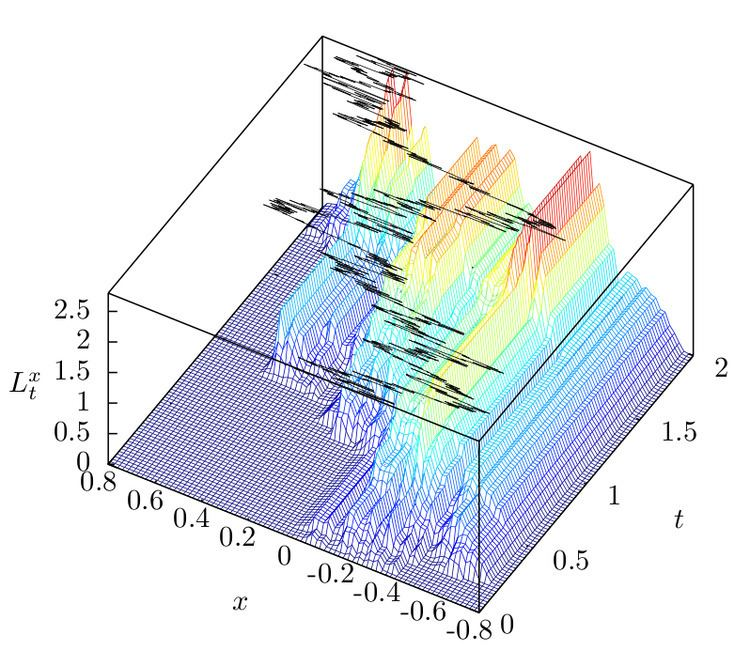# Local time (mathematics)

Updated onIn the mathematical theory of stochastic processes, local time is a stochastic process associated with diffusion processes such as Brownian motion, that characterizes the amount of time a particle has spent at a given level. Local time appears in various stochastic integration formulas, such as Tanaka's formula, if the integrand is not sufficiently smooth. It is also studied in statistical mechanics in the context of random fields.

## Formal definition

For a real valued diffusion process ( B s ) s 0 , the local time of B at the point x is the stochastic process

L x ( t ) = 0 t δ ( x B s ) d s ,

where δ is the Dirac delta function. It is a notion invented by Paul Lévy. The basic idea is that L x ( t ) is a (rescaled) measure of how much time B s has spent at x up to time t . It may be written as

L x ( t ) = lim ε 0 1 2 ε 0 t 1 { x ε < B s < x + ε } d s ,

which explains why it is called the local time of B at x . For a discrete state-space process ( X s ) s 0 , the local time can be expressed more simply as

L x ( t ) = 0 t 1 { x } ( X s ) d s .

## Tanaka's formula

Tanaka's formula provides a definition of local time for an arbitrary continuous semimartingale ( X s ) s 0 on R :

L x ( t ) = | X t x | | X 0 x | 0 t ( 1 ( 0 , ) ( X s x ) 1 ( , 0 ] ( X s x ) ) d X s , t 0.

A more general form was proven independently by Meyer and Wang; the formula extends Itô's lemma for twice differentiable functions to a more general class of functions. If F : R R is absolutely continuous with derivative F , which is of bounded variation, then

F ( X t ) = F ( X 0 ) + 0 t F ( X s ) d X s + 1 2 L x ( t ) d F ( x ) ,

where F is the left derivative.

If X is a Brownian motion, then for any α ( 0 , 1 / 2 ) the field of local times L = ( L x ( t ) ) x R , t 0 has a modification which is a.s. Hölder continuous in x with exponent α , uniformly for bounded x and t . In general, L has a modification that is a.s. continuous in t and cadlag in x .

Tanaka's formula provides the explicit Doob–Meyer decomposition for the one-dimensional reflecting Brownian motion, ( | B s | ) s 0 .

## Ray–Knight theorems

The field of local times L t = ( L t x ) x E associated to a stochastic process on a space E is a well studied topic in the area of random fields. Ray–Knight type theorems relate the field Lt to an associated Gaussian process.

In general Ray–Knight type theorems of the first kind consider the field Lt at a hitting time of the underlying process, whilst theorems of the second kind are in terms of a stopping time at which the field of local times first exceeds a given value.

## First Ray–Knight theorem

Let (Bt)t ≥ 0 be a one-dimensional Brownian motion started from B0 = a > 0, and (Wt)t≥0 be a standard two-dimensional Brownian motion W0 = 0 ∈ R2. Define the stopping time at which B first hits the origin, T = inf { t 0 : B t = 0 } . Ray and Knight (independently) showed that

where (Lt)t ≥ 0 is the field of local times of (Bt)t ≥ 0, and equality is in distribution on C[0, a]. The process |Wx|2 is known as the squared Bessel process.

## Second Ray–Knight theorem

Let (Bt)t ≥ 0 be a standard one-dimensional Brownian motion B0 = 0 ∈ R, and let (Lt)t ≥ 0 be the associated field of local times. Let Ta be the first time at which the local time at zero exceeds a > 0

T a = inf { t 0 : L t 0 > a } .

Let (Wt)t ≥ 0 be an independent one-dimensional Brownian motion started from W0 = 0, then

Equivalently, the process ( L T a x ) x 0 (which is a process in the spatial variable x ) is equal in distribution to the square of a 0-dimensional Bessel process, and as such is Markovian.

## Generalized Ray–Knight theorems

Results of Ray–Knight type for more general stochastic processes have been intensively studied, and analogue statements of both (1) and (2) are known for strongly symmetric Markov processes.

Similar Topics